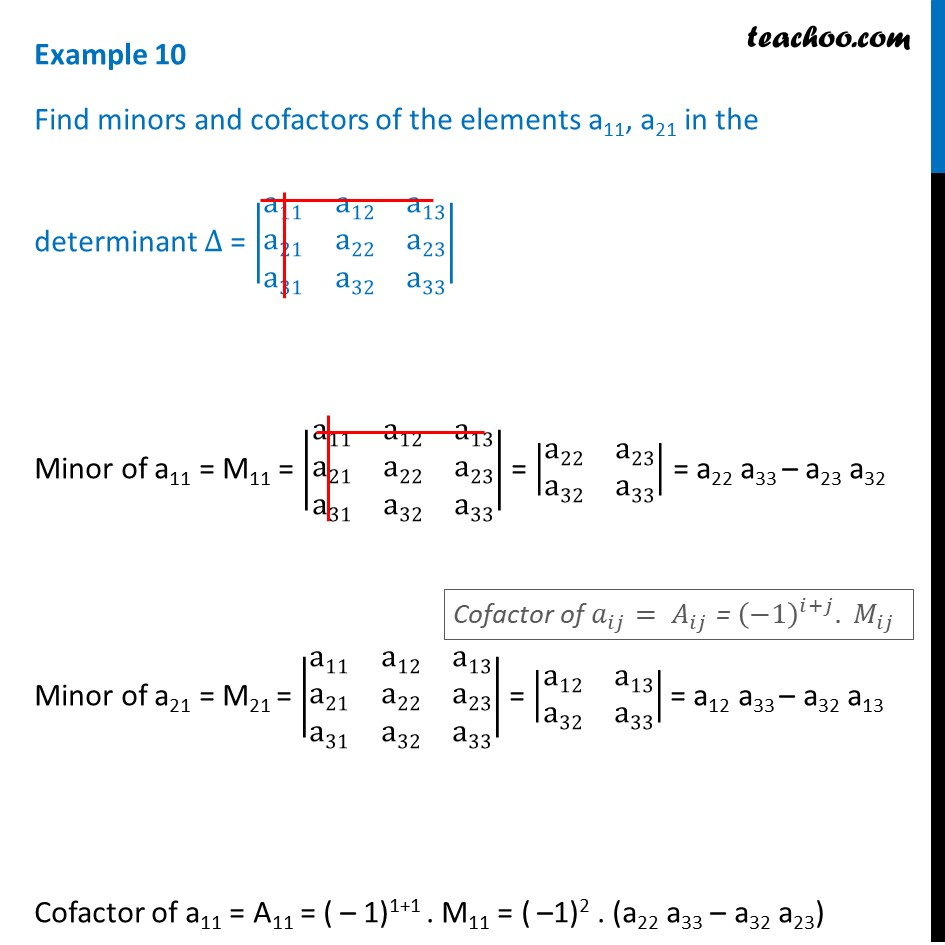Examples

Chapter 4 Class 12 Determinants
Serial order wiseLearn in your speed, with individual attention - Teachoo Maths 1-on-1 Class

### Transcript

Example 10 Find minors and cofactors of the elements a11, a21 in the determinant ∆ = |■8(a11&a12&a13@a21&a22&a23@a31&a32&a33)| Minor of a11 = M11 = |■8(a11&a12&a13@a21&a22&a23@a31&a32&a33)| = |■8(a22&a23@a32&a33)| = a22 a33 – a23 a32 Minor of a21 = M21 = |■8(a11&a12&a13@a21&a22&a23@a31&a32&a33)| = |■8(a12&a13@a32&a33)| = a12 a33 – a32 a13 Cofactor of a11 = A11 = ( – 1)1+1 . M11 = ( –1)2 . (a22 a33 – a32 a23) = a22 a33 – a32 a23 Cofactor of a21 = A21 = ( – 1)2+1 . M21= ( – 1)3 . (a12 a33 – a32 a13) = ( – 1) . (a12 a33 – a32 a13) = a32 a13 – a12 a33